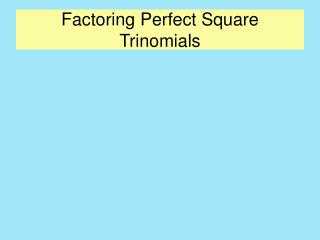DownloadDownload PresentationFactoring Perfect Square Trinomials

# Factoring Perfect Square Trinomials

Télécharger la présentation## Factoring Perfect Square Trinomials

- - - - - - - - - - - - - - - - - - - - - - - - - - - E N D - - - - - - - - - - - - - - - - - - - - - - - - - - -
##### Presentation Transcript

1. Factoring Perfect Square Trinomials

2. Factoring Perfect Square Trinomials What is a perfect square? If a number is squared the result is a perfect square. Example 22=4 4 is a perfect square. Other examples: 32=9 or 42=16 9 is a perfect square. 16 is a perfect square.

3. Factoring Perfect Square Trinomials Here is a list of the perfect squares for the numbers 1-30. 12=1 112=121 212=441 22=4 122=144 222=484 32=9 132=169 232=529 42=16 142=196 242=576 52=25 152=225 252=625 62=36 162=256 262=676 72=49 172=289 272=729 82=64 182=324 282=784 92=81 192=361 292=841 102=100 202=400 302=900

4. Factoring Perfect Square Trinomials When a variable is raised to an even power it is a perfect square. Example: (x)(x)= x2 x2 is a perfect square. (x3)(x3)= x6 or (x5)(x5)= x10 x6 and x10 are both perfect squares.

5. Factoring Perfect Square Trinomials If a number or a variable is a perfect square the square root of the quantity is the number or variable that was squared to get the perfect square. Example: Square 9. 9x9 = 81 81 is the perfect square. 9 is the square root of 81. Example: Square x3 (x3) (x3) = x6 or (x3)2 = x6 x6is the perfect square. x3 is the square root of x6

6. Factoring Perfect Square Trinomials • Now we are ready to understand the term- perfect square trinomial. • The trinomial that results from squaring a binomial is a perfect square trinomial. • Example: (x+7)2 = x2+14x+49 • x2+14x+49 is a perfect square trinomial. • We know that a perfect square trinomial always results when a binomial is squared. • The reverse is also true. • When we factor a perfect square trinomial the result is always a squared binomial.

7. Factoring Perfect Square Trinomials • Here are few examples: • Factor: x2+10x+25 • Result: (x+5)2 • Check by multiplying • x2+10x+25 • Factor: x2+2xy+y2 • (x+y) 2 • Check by multiplying. • x2+2xy+y2

8. Factoring Perfect Square Trinomials • Not all trinomials are perfect square trinomials. • How do we recognize that a trinomial is a perfect square trinomial. • The first and last terms of the trinomial must be perfect squares and must be positive. • Example: x2+10x+25 • What about the middle term? +10x • Take the square root of the first term x2 and get x. • Take the square root of the last term +25 and get 5. • Multiply (5)(x) and double the result. 10x. That is your middle term. • Two times the product of the square roots of the first and last terms will give the middle term.

9. Factoring Perfect Square Trinomials • Here are some examples of trinomials that are perfect square trinomials. • 4x2 -20x +25 • 2x • 5 • (2x- 5)2 • 9x2 - 48xy + 64y2 • 3x • 8y • (3x-8y)2 • 2x3 +20x2y+50xy2 • Factor out the GCF • 2x(x2+10xy+25y2) • 2x • x • 5y • 2x(x+5y)2

10. Factoring Perfect Square Trinomials Here are some examples that are not perfect square trinomials. x2+10x-25 The last term is not positive. x2+2xy+2y2 The 2 in the last term is not a perfect square. 4x2-10xy+25y2 The square root of the first term is 2x. The square root of the last term 5y. 2(2x)(5y)= 20xy 20xy = 10xy 4x2-16xy+8y2 There is a common factor of four. 4(x2- 4xy + 2y2) The last term of the trinomial is not a perfect square because the 2 in the last term is not perfect square. To get more help go to the tutorial Practice- Factoring Perfect Square Trinomials